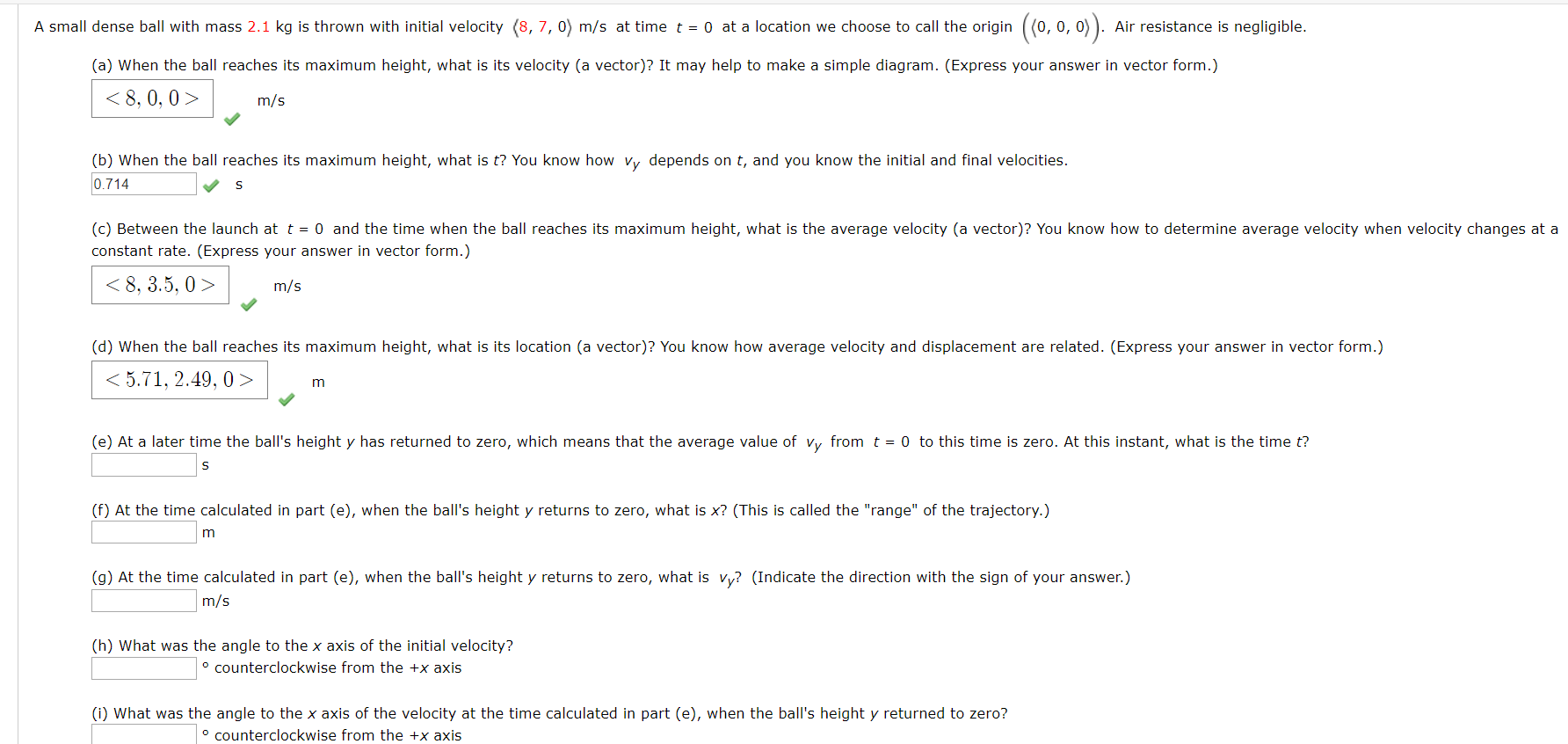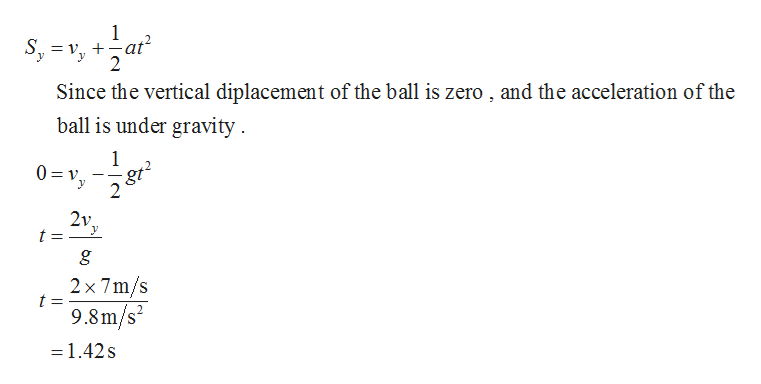# (0, 0, 0)A small dense ball with mass 2.1 kg is thrown with initial velocity (8, 7, 0) m/s at time t = 0 at a location we choose to call the originAir resistance is negligible.(a) When the ball reaches its maximum height, what is its velocity (a vector)? It may help to make a simple diagram. (Express your answer in vector form.)m/s(b) When the ball reaches its maximum height, what is t? You know how vy depends on t, and you know the initial and final velocities.0.714(c) Between the launch at t = 0 and the time when the ball reaches its maximum height, what is the average velocity (a vector)? You know how to determine average velocity when velocity changes at aconstant rate. (Express your answer in vector form.)8, 3.5, 0>m/s(d) When the ball reaches its maximum height, what is its location (a vector)? You know how average velocity and displacement are related. (Express your answer in vector form.)5.71, 2.49, 0 >m(e) At a later time the ball's height y has returned to zero, which means that the average value of vy from t = 0 to this time is zero. At this instant, what is the time t?S(f) At the time calculated in part (e), when the ball's height y returns to zero, what is x? (This is called the "range" of the trajectory.)m(g) At the time calculated in part (e), when the ball's height y returns to zero, what is vy? (Indicate the direction with the sign of your answer.)m/s(h) What was the angle to the x axis of the initial velocity?oCounterclockwise from the +x axis(i) What was the angle to the x axis of the velocity at the time calculated in part (e), when the ball's height y returned to zero?counterclockwise from the +x axis

Question
37 views

How would you solve for the last four parts?help_outlineImage Transcriptionclose(0, 0, 0) A small dense ball with mass 2.1 kg is thrown with initial velocity (8, 7, 0) m/s at time t = 0 at a location we choose to call the origin Air resistance is negligible. (a) When the ball reaches its maximum height, what is its velocity (a vector)? It may help to make a simple diagram. (Express your answer in vector form.) <8, 0, 0> m/s (b) When the ball reaches its maximum height, what is t? You know how vy depends on t, and you know the initial and final velocities. 0.714 (c) Between the launch at t = 0 and the time when the ball reaches its maximum height, what is the average velocity (a vector)? You know how to determine average velocity when velocity changes at a constant rate. (Express your answer in vector form.) 8, 3.5, 0> m/s (d) When the ball reaches its maximum height, what is its location (a vector)? You know how average velocity and displacement are related. (Express your answer in vector form.) 5.71, 2.49, 0 > m (e) At a later time the ball's height y has returned to zero, which means that the average value of vy from t = 0 to this time is zero. At this instant, what is the time t? S (f) At the time calculated in part (e), when the ball's height y returns to zero, what is x? (This is called the "range" of the trajectory.) m (g) At the time calculated in part (e), when the ball's height y returns to zero, what is vy? (Indicate the direction with the sign of your answer.) m/s (h) What was the angle to the x axis of the initial velocity? oCounterclockwise from the +x axis (i) What was the angle to the x axis of the velocity at the time calculated in part (e), when the ball's height y returned to zero? counterclockwise from the +x axis fullscreen
check_circle

Step 1

Write the expression for vertical displacement of the small dense ball, and simplify the equation to get t then Substitute 7m/s for vy, and 9.8m/s2 for g in the below equation.help_outlineImage Transcriptionclose1 = v, +-at 2 Since the vertical diplacement of the ball is zero , and the acceleration of the ball is under gravity 1 0 v gt 2 2v t = g 2x 7m/s 9.8m/s2 =1.42s fullscreen
Step 2

(f) Write the expression for range and substitute 8m/s for vx, and 1.42s for t in the below equation.

Step 3

(g) Write the expression for velocity a...

### Want to see the full answer?

See Solution

#### Want to see this answer and more?

Solutions are written by subject experts who are available 24/7. Questions are typically answered within 1 hour.*

See Solution
*Response times may vary by subject and question.
Tagged in
SciencePhysics

### Kinematics